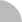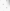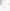# 臺灣博碩士論文加值系統

(44.201.72.250) 您好！臺灣時間：2023/10/04 18:15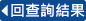:::

### 詳目顯示

: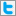Twitter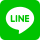•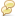被引用:0
•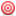點閱:268
•評分: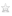•下載:6
•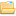書目收藏:0
 On hypoellipticity of the @b operator on weakly pseudoconvex CR manifold Let D Cn, n 2, be a CR manifold with smooth boundary, and let r be a smooth defining function for D. Hence, the set {Lk = @r @zn @ @zk − @r @zk @ @zn | k = 1, 2, · · · , n − 1} forms a global basis for the space of tangential (1,0) vector fields on the boundary bD. If D is strongly pseudoconvex, then bD is strongly pseudoconvex CR manifold. For example, we consider the Siegel upper half space = {(z0, zn) 2 Cn | Imzn > |z0|2} Cn. The set {Lk = @ @zk + 2izk @ @zn | k = 1, · · · , n − 1} forms a global basis for the space of tangential (1,0) vector fields on the boundary b . If we choose T = −2i @ @t , then the Levi matrix is the identity matrix. Moreover, the surface b is a strictly pseudoconvex CR manifold. As coordinates for the surface we use Hn = Cn−1 × R 3 (z0, t) 7! (z0, t + i|z0|2); the vector fields pull back to Zk = @ @zk + izk @ @t . The Heisenberg group Hn is a strictly pseudoconvex CR manifold with type (1,0) vector fields spanned by Z1, . . . ,Zn−1. Then we can get b = @b@ b+@ b@b is hypoelliptic on Hn for (0, q)-forms when 1 q n−1. But hypoellipticity of @ b does not always hold on a pseudoconvex CR manifold M which is not strongly pseudoconvex. For example, we consider the domain D = {(z1, z2) 2 C2 | Imz2 > [ReZ1]m,m 4 is even}. Set M to be the boundary bD, and the tangential (1,0) vector field on M is Z = @ @z1 + im 2 xm−1 1 @ @t , where x = Rez1 and z2 = t + is. Let S((z, t); (w, s)) be the Szeg¨o projection from L2(C × R) onto the kernel of Z. Define the distribution K(z, t) = S((z, t); (0, 0)). Then we can prove that K is not analytic away from 0. In the case M = {(z1, z2, z3) 2 C3 | Imz3 = [Rez1]m +|z2|2,m 4 is even}, the tangential (1,0) vector fields are spanned by Z1 = @ @z1 +im 2 xm−1 1 @ @t , and Z2 = @ @z2 +iz2 @ @t . Similarly, the Szeg¨o projection S is the orthogonal projection from L2 onto {f 2 L2 | Z1f = Z2 = 0}. Let J(z1, z2, t) = S((z1, z2, t), (0, 0, 0)). Then we can prove that J is not analytic away from 0, too. Now, we consider M = {(z1, z2) 2 C2 | Imz2 = xm,m 4 is even}. We prove the failure of @b to be analytic hypoelliptic on M directly. We examine f(x) = e2(x+xm) Rx −1 e−4(s+sm)ds , and define f(x + iy, t) = Z 0 −1 e−2ite−2i||1/myf(||1/mx) d . A calculation shows @b@ bf = 0, but @ bf(0 − i, t) is not analytic at t = 0. 1
 On hypoellipticity of the @b operator on weakly pseudoconvex CR manifold Let D Cn, n 2, be a CR manifold with smooth boundary, and let r be a smooth defining function for D. Hence, the set {Lk = @r @zn @ @zk − @r @zk @ @zn | k = 1, 2, · · · , n − 1} forms a global basis for the space of tangential (1,0) vector fields on the boundary bD. If D is strongly pseudoconvex, then bD is strongly pseudoconvex CR manifold. For example, we consider the Siegel upper half space = {(z0, zn) 2 Cn | Imzn > |z0|2} Cn. The set {Lk = @ @zk + 2izk @ @zn | k = 1, · · · , n − 1} forms a global basis for the space of tangential (1,0) vector fields on the boundary b . If we choose T = −2i @ @t , then the Levi matrix is the identity matrix. Moreover, the surface b is a strictly pseudoconvex CR manifold. As coordinates for the surface we use Hn = Cn−1 × R 3 (z0, t) 7! (z0, t + i|z0|2); the vector fields pull back to Zk = @ @zk + izk @ @t . The Heisenberg group Hn is a strictly pseudoconvex CR manifold with type (1,0) vector fields spanned by Z1, . . . ,Zn−1. Then we can get b = @b@ b+@ b@b is hypoelliptic on Hn for (0, q)-forms when 1 q n−1. But hypoellipticity of @ b does not always hold on a pseudoconvex CR manifold M which is not strongly pseudoconvex. For example, we consider the domain D = {(z1, z2) 2 C2 | Imz2 > [ReZ1]m,m 4 is even}. Set M to be the boundary bD, and the tangential (1,0) vector field on M is Z = @ @z1 + im 2 xm−1 1 @ @t , where x = Rez1 and z2 = t + is. Let S((z, t); (w, s)) be the Szeg¨o projection from L2(C × R) onto the kernel of Z. Define the distribution K(z, t) = S((z, t); (0, 0)). Then we can prove that K is not analytic away from 0. In the case M = {(z1, z2, z3) 2 C3 | Imz3 = [Rez1]m +|z2|2,m 4 is even}, the tangential (1,0) vector fields are spanned by Z1 = @ @z1 +im 2 xm−1 1 @ @t , and Z2 = @ @z2 +iz2 @ @t . Similarly, the Szeg¨o projection S is the orthogonal projection from L2 onto {f 2 L2 | Z1f = Z2 = 0}. Let J(z1, z2, t) = S((z1, z2, t), (0, 0, 0)). Then we can prove that J is not analytic away from 0, too. Now, we consider M = {(z1, z2) 2 C2 | Imz2 = xm,m 4 is even}. We prove the failure of @b to be analytic hypoelliptic on M directly. We examine f(x) = e2(x+xm) Rx −1 e−4(s+sm)ds , and define f(x + iy, t) = Z 0 −1 e−2ite−2i||1/myf(||1/mx) d . A calculation shows @b@ bf = 0, but @ bf(0 − i, t) is not analytic at t = 0. 1
 11
  A. Nagel, Vector fields and nonisotropic metrics, in BeijingLectures in Harmonic Analysis, Ann. of Math. Studies 112,Princeton University Press, Princeton, N.J. (1986),241-306. A. Nagel and E.M. Stein, Lectures on pseudo-dierential operators:Regularity theorems and applications to non-ellipticproblems, Math. Notes 34, Princeton University Press, Princeton,N.J. (1979). D. Geller, Analytic pseudodierential operators for Heisenberggroup and local solvability, Math. Notes 37, Princeton UniversityPress, Princeton, N.J. (1990). D.S. Tartako, Local analytic hypoellipticity for b on nondegenerateCauchy-Riemann manifolds, Proc. Nat. Acad.Sci. U.S.A. 75 (1978), 3027-3028. F. Treves, Analytic hypoellipticity of a class of pseudodierentialoperators with double characteristics and applications tothe @-Neumann problem, Comm. in P.D.E. 3 (1978), 475-642. G.B. Folland and E.M. Stein, Estimates for the @b complex andanalysis on the Heisenberg group, Comm. Pure and AppliedMath. 27 (1974), 429-522. M. Christ and D. Geller, Counterexamples to analytic hypoellipticityfor domains of finite type, Ann. Math. 135 (1992),511-566. S.C. Chen and M.C. Shaw, Partial dierential equations inseveral complex variables, Studies in Advanced Math. 19(2001).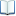電子全文推文當script無法執行時可按︰推文 網路書籤當script無法執行時可按︰網路書籤 推薦當script無法執行時可按︰推薦 評分當script無法執行時可按︰評分 引用網址當script無法執行時可按︰引用網址 轉寄當script無法執行時可按︰轉寄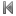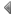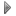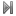top
 無相關論文

 無相關期刊

 1 圖譜剩餘定理及其應用 2 一個四階半線性拋物型方程的零邊界控制 3 N+1維複數空間中實值超曲面的局部行為 4 李朝世宗時期的朝鮮算學 5 中國1368-1806年間的勾股術發展之研究 6 多維phi係數的齊性檢定研究 7 變異型態的最小最大定理 8 一個非線性消失性問題相似解的存在性 9 國三學生突破因附圖造成之論證障礙的學習歷程之研究 10 於動態幾何環境下國中生動態心像建構與幾何推理之研究 11 三位學生教師數學教學概念轉變歷程的個案研究 12 UndecidabilityofFinitenessConjectureforgeneralizedspectralradius 13 有限體上的戴凡波問題 14 關於變分不等式的輔助問題原理 15 平面二次與三次曲線的希爾伯特方程式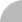簡易查詢 | 進階查詢 | 熱門排行 | 我的研究室